• 一、分类算法中的损失函数在分类算法中,损失函数通常可以表示成损失项和正则项的和，即如下的形式： J(w)=∑iL(mi(w))+λR(w)其中，L(mi(w))L(mi(w))为损失项，R(w)R(w)为正则项。mimi的具体形式如下： mi=y(i)fw...
https://blog.csdn.net/wyisfish/article/details/79255211https://blog.csdn.net/wjc1182511338/article/details/76793598https://blog.csdn.net/wenzishou/article/details/77618992指数损失函数（Adaboost）学过Adaboost算法的人都知道，它是前向分步加法算法的特例，是一个加和模型，损失函数就是指数函数。在Adaboost中，经过m此迭代之后，可以得到Adaboost每次迭代时的目的是为了找到最小化下列式子时的参数而指数损失函数(exp-loss）的标准形式如下可以看出，Adaboost的目标式子就是指数损失，在给定n个样本的情况下，Adaboost的损失函数为：指数损失函数（Adaboost）学过Adaboost算法的人都知道，它是前向分步加法算法的特例，是一个加和模型，损失函数就是指数函数。在Adaboost中，经过m此迭代之后，可以得到:Adaboost每次迭代时的目的是为了找到最小化下列式子时的参数 和G：而指数损失函数(exp-loss）的标准形式如下可以看出，Adaboost的目标式子就是指数损失，在给定n个样本的情况下，Adaboost的损失函数为：Hinge损失函数（SVM）在机器学习算法中，hinge损失函数和SVM是息息相关的。在线性支持向量机中，最优化问题可以等价于下列式子：下面来对式子做个变形，令：于是，原式就变成了：如若取，式子就可以表示成：可以看出，该式子与下式非常相似：前半部分中的就是hinge损失函数，而后面相当于L2正则项。决策树损失函数设决策树的子节点集合为T，t是T中的一个元素，该叶节点有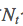个样本，其中k类的样本有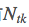个，共K个分类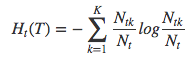则损失函数可以定义为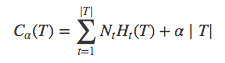右边第一项表示误差大小，第二项表示模型的复杂度，也就是用叶节点表示，防止过拟化。
展开全文• 0-1损失函数 平方损失函数（最小二乘法） 最小二乘法是线性回归的一种，OLS将问题转化成了一个凸优化问题。在线性回归中，它假设样本和噪声都服从高斯分布（为什么假设成高斯分布呢？其实这里隐藏了一个小知识...
参考：http://blog.csdn.net/google19890102/article/details/50522945
0-1损失函数平方损失函数（最小二乘法）
最小二乘法是线性回归的一种，OLS将问题转化成了一个凸优化问题。在线性回归中，它假设样本和噪声都服从高斯分布（为什么假设成高斯分布呢？其实这里隐藏了一个小知识点，就是中心极限定理，可以参考
简单，计算方便；
欧氏距离是一种很好的相似性度量标准；
在不同的表示域变换后特征性质不变。
平方损失（Square loss）的标准形式如下：当样本个数为n时，此时的损失函数变为：Y-f(X)表示的是残差，整个式子表示的是残差的平方和，而我们的目的就是最小化这个目标函数值（注：该式子未加入正则项），也就是最小化残差的平方和（residual sum of squares，RSS）。

而在实际应用中，通常会使用均方差（MSE）作为一项衡量指标，公式如下：Log损失函数
Log损失是0-1损失函数的一种代理函数，Log损失的具体形式如下：运用Log损失的典型分类器是Logistic回归算法。

Hinge损失函数
Hinge损失是0-1损失函数的一种代理函数，Hinge损失的具体形式如下：运用Hinge损失的典型分类器是SVM算法。

指数损失
指数损失是0-1损失函数的一种代理函数，指数损失的具体形式如下：感知损失
感知损失是Hinge损失的一个变种，感知损失的具体形式如下：运用感知损失的典型分类器是感知机算法。

转载于:https://www.cnblogs.com/home123/p/7358780.html
展开全文• 机器学习损失函数小结概述损失函数、代价函数、目标函数的区别与联系分类问题中的损失函数0-1损失函数Hinge损失函数Logistic损失函数交叉熵损失函数回归问题中的损失函数平方损失函数绝对损失函数Huber损失函数 ...
机器学习损失函数小结概述损失函数、代价函数、目标函数的区别与联系分类问题中的损失函数0-1损失函数Hinge损失函数Logistic损失函数交叉熵损失函数回归问题中的损失函数平方损失函数绝对损失函数Huber损失函数
概述
机器学习算法实际上就是模型表征、优化和评估的过程，其中，模型评估决定了后续的优化算法及模型改进的走向，而衡量模型评估的指标就是损失函数。参考李航老师的《统计学习方法》关于损失函数的描述：

监督学习问题是在假设空间$\mathcal{F}$中选取模型$f$作为决策函数，对于给定的输入$X$，由$f(X)$给出相应的输出$Y$，这个输出的预测值$f(X)$与真实值$Y$可能一致也可能不一致，用一个损失函数（loss function）或代价函数（cost function）来度量预测错误的程度。损失函数是$f(X)$和$Y$的非负实值函数，记作L(Y,f(X))​。 损失函数用来评价模型的预测值和真实值不一样的程度，损失函数越小，通常模型的性能越好。不同的模型用的损失函数一般也不一样。 损失函数分为经验风险损失函数和结构风险损失函数，经验风险损失函数指预测结果和实际结果的差别，结构风险损失函数是指经验风险损失函数加上正则项。 由于模型的输入、输出$(X,Y)$是随机变量，遵循联合分布$P(X,Y)$，所以损失函数的期望为： KaTeX parse error: No such environment: align* at position 8: \begin{̲a̲l̲i̲g̲n̲*̲}̲ R_{exp}(f)&=E_… 这是理论上模型$f(X)$关于联合分布$P(X,Y)$的平均意义下的损失，称为风险函数（risk function）或期望损失（expected loss）。学习的目标就是选择期望风险最小的模型，但由于联合分布$P(X,Y)$未知，$R_{exp}(f)$不能直接计算。 给定一个训练数据集 $T=\left\{ (x_1,y_1),(x_2,y_2),...,(x_N,y_N) \right\}$ 模型$f(X)$关于训练数据集的平均损失称为经验风险（empirical risk）或经验损失（empirical loss），记作$R_{emp}$： $R_{emp}(f)=\frac{1}{N}\sum_{i=1}^NL(y_i,f(x_i))\tag2$ 期望风险$R_{exp}$是模型关于联合分布的期望损失，经验风险$R_{emp}$是模型关于训练数据集的平均损失。根据大数定律，当样本容量$N$趋于无穷时，经验风险$R_{emp}$趋于期望风险$R_{exp}$。所以，可以利用经验风险估计期望风险。 损失函数、代价函数、目标函数的区别与联系 损失函数(loss function)通常是针对单个训练样本而言； 代价函数(cost function)通常是针对整个数据集（或一个mini-batch）； 目标函数(objective function)表示任意希望被优化的函数。 分类问题中的损失函数 为了刻画模型输出与样本标签的匹配程度，定义损失函数$L(\bullet,\bullet$)$:$$Y×Y$ $\to$ $R$，$L(f(x_i,\theta),y_i)$越小，表明模型在该样本点匹配得越好。 0-1损失函数 对于二分类问题，$Y=\lbrace-1，1 \rbrace$，我们希望$signf(x_i,\theta)=y_i$，最自然的损失函数为0-1损失，即: $L_{0-1}(f,y)=1_{fy \leq 0} \tag{3}$ 其中，$1_p$为指示函数（Indicator Function），当且仅当P为真时取值为1，否则取值为0。该损失函数能直观地刻画分类的错误率，但是由于其非凸、非光滑的特点，使得算法很难直接对该函数进行优化。 Hinge损失函数 0-1损失函数的一个代理损失函数为Hinge损失函数： $L_{hinge}(f,y)=max\lbrace0,1-fy\rbrace \tag{4}$ Hinge损失函数是0-1损失函数相对紧的凸上界，且当$fy \geq 1$时，该函数不对其做任何惩罚。由于Hinge损失在$fy=1$处不可导，因此不能用梯度下降法进行优化，而是用次梯度下降法（Subgra- dient Descent Method）。 Logistic损失函数 0-1损失函数的另一个代理损失函数为Logistic损失函数： $L_{Logistic}(f,y)=log_2(1+exp(-fy)) \tag{5}$ Logistic损失函数也是0-1损失函数的凸上界，且该函数处处光滑，因此可以用梯度下降法进行优化。但是，该损失函数对所有的样本点都有所惩罚，因此对异常值相对更敏感一些。 交叉熵损失函数 当预测值$f\in[-1,1]$时， 另一个常用的代理损失函数为交叉熵（Cross Entroy）损失函数： $L_{crossentroy}(f,y)=-log_2(\frac{1+fy}{2}) \tag{6}$ 交叉熵损失函数也是0-1损失函数的光滑凸上界。这四种损失函数的曲线如下图所示：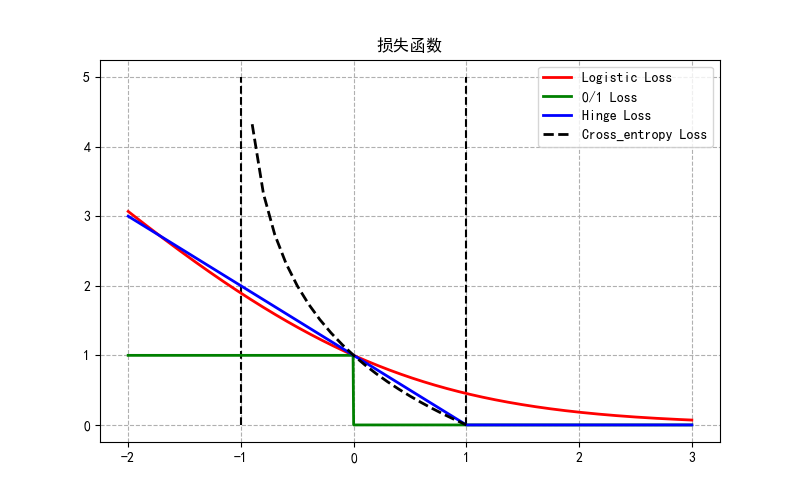图1 二分类问题的损失函数 源码如下： import numpy as np import math import matplotlib.pyplot as plt plt.rcParams['font.sans-serif']=['SimHei'] plt.rcParams['axes.unicode_minus'] = False plt.figure(figsize=(8, 5)) x = np.linspace(start=-2, stop=3,num =1001,dtype=np.float) x1=np.linspace(start=-1, stop=1,num =21,dtype=np.float) logi = np.log(1 + np.exp(-x))/math.log(2) cross_entropy = -np.log2((1+x1)/2) boost = np.exp(-x) y_01 = x < 0 y_hinge = 1.0 - x y_hinge[y_hinge < 0] = 0 #S_values = [x=3] plt.plot(x, logi, 'r-', mec='k', label='Logistic Loss', lw=2) plt.plot(x, y_01, 'g-', mec='k', label='0/1 Loss', lw=2) plt.plot(x, y_hinge, 'b-',mec='k', label='Hinge Loss', lw=2) #plt.plot(x, boost, 'm--',mec='k', label='Adaboost Loss',lw=2) plt.plot(x1, cross_entropy, 'k--',mec='k', label='Cross_entropy Loss',lw=2) plt.vlines(-1, 0,5,colors = "k", linestyles = "dashed") plt.vlines(1, 0,5,colors = "k", linestyles = "dashed") plt.grid(True, ls='--') plt.legend(loc='upper right') plt.title('损失函数') plt.show()  回归问题中的损失函数 对于回归问题，$Y=R$，我们希望$f(x_i,\theta) \approx y_i$，常用的损失函数包括如下： 平方损失函数 $L_{squre}(f,y)=(f-y)^2 \tag{5}$ 平方损失函数时光滑函数，能够用梯度下降法来进行优化。然而，当预测值距离真实值越远时，平方损失函数的惩罚力度越大，因此它对异常类比较敏感。 绝对损失函数 为了解决平方损失函数对异常类比较敏感的问题，可采用绝对损失函数： $L_{absoulte}(f,y)= \vert{f-y}\vert \tag{6}$ 绝对损失函数相当于在做中值回归，相比做均值回归的平方损失函数，绝对损失函数对异常点更鲁棒一些。但是，绝对损失函数在$f=y$处无法求导数。 Huber损失函数 综合考虑可导性和对异常点的鲁棒性，可以采用Huber损失函数： $L_{Huber}(f,y)=\left\{\begin{matrix} (f-y)^2, &\vert{f-y}\vert^2\leq \delta \\ 2\delta\vert{f-y}\vert-\delta^2,& \vert{f-y}\vert \gt \delta \end{matrix}\right.\tag{7}$ Huber损失函数在$\vert{f-y}\vert$较小时为平方损失，在$\vert{f-y}\vert$较大时为线性损失，处处可导，且对异常点鲁棒。这三种损失函数的曲线如图2所示：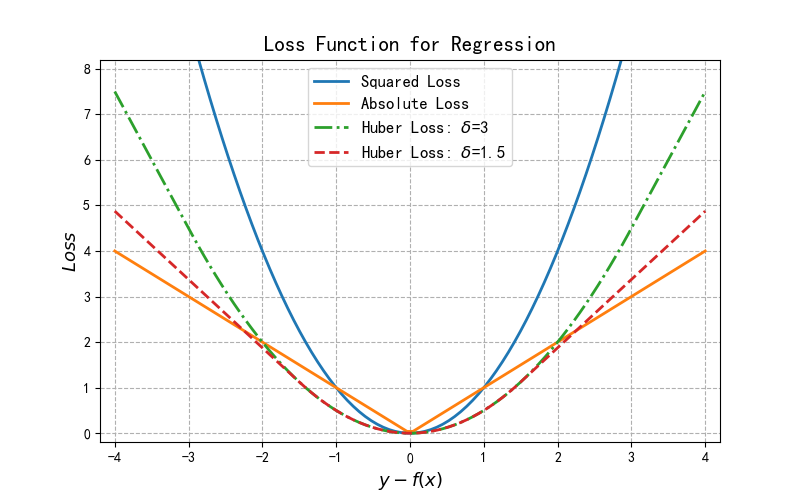图2 回归问题的损失函数 源码如下： import numpy as np import matplotlib.pyplot as plt plt.rcParams['font.sans-serif']=['SimHei'] plt.rcParams['axes.unicode_minus'] = False plt.figure(figsize=(8, 5)) def huber(res, delta): res = abs(res) return (res<=delta)*(res**2/2) + (res>delta)*(delta*res-delta**2/2) plt.axis([-4.2,4.2,-0.2,8.2]) x = np.linspace(-4,4,100) plt.plot(x, x**2, label="Squared Loss", lw=2) plt.plot(x,np.where(x>=0,x,-x), label="Absolute Loss", lw=2) plt.plot(x,huber(x, 3), label="Huber Loss:\delta$={}".format(3),lw=2, linestyle="-.") plt.plot(x,huber(x, 1.5), label="Huber Loss:$\delta$={}".format(1.5),lw=2,linestyle="--") plt.grid(True, ls='--') plt.legend(loc='upper center', fontsize=12) plt.xlabel("$y-f(x)$",fontsize=13) plt.ylabel("$Loss\$",fontsize=13)
plt.title("Loss Function for Regression",fontsize=15)



展开全文• 常见机器学习损失函数（Loss Function） 　损失函数（loss function）是用来估量模型的预测值f(x)与真实值Y的不一致程度，它是一个非负实值函数,通常使用L(Y, f(x))来表示，损失函数越小，模型的鲁棒性就越好...


常见机器学习损失函数（Loss Function）

损失函数（loss function）是用来估量模型的预测值f(x)与真实值Y的不一致程度，它是一个非负实值函数,通常使用L(Y, f(x))来表示，损失函数越小，模型的鲁棒性就越好。损失函数是经验风险函数的核心部分，也是结构风险函数重要组成部分。模型的结构风险函数包括了经验风险项和正则项，通常可以表示成如下式子：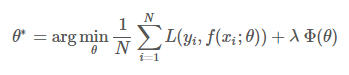其中，前面的均值函数表示的是经验风险函数，L代表的是损失函数，后面的Φ是正则化项（regularizer）或者叫惩罚项（penalty term），它可以是L1，也可以是L2，或者其他的正则函数。整个式子表示的意思是找到使目标函数最小时的θ值。下面主要列出几种常见的损失函数。
理解：损失函数旨在表示出logit和label的差异程度，不同的损失函数有不同的表示意义，也就是在最小化损失函数过程中，logit逼近label的方式不同，得到的结果可能也不同。
一般情况下，softmax和sigmoid使用交叉熵损失（logloss），hingeloss是SVM推导出的，hingeloss的输入使用原始logit即可。
一、LogLoss对数损失函数（逻辑回归，交叉熵损失）
有些人可能觉得逻辑回归的损失函数就是平方损失，其实并不是。平方损失函数可以通过线性回归在假设样本是高斯分布的条件下推导得到，而逻辑回归得到的并不是平方损失。在逻辑回归的推导中，它假设样本服从伯努利分布（0-1分布），然后求得满足该分布的似然函数，接着取对数求极值等等。而逻辑回归并没有求似然函数的极值，而是把极大化当做是一种思想，进而推导出它的经验风险函数为：最小化负的似然函数（即max F(y, f(x)) —> min -F(y, f(x)))。从损失函数的视角来看，它就成了log损失函数了。
log损失函数的标准形式：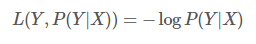刚刚说到，取对数是为了方便计算极大似然估计，因为在MLE（最大似然估计）中，直接求导比较困难，所以通常都是先取对数再求导找极值点。损失函数L(Y, P(Y|X))表达的是样本X在分类Y的情况下，使概率P(Y|X)达到最大值（换言之，就是利用已知的样本分布，找到最有可能（即最大概率）导致这种分布的参数值；或者说什么样的参数才能使我们观测到目前这组数据的概率最大）。因为log函数是单调递增的，所以logP(Y|X)也会达到最大值，因此在前面加上负号之后，最大化P(Y|X)就等价于最小化L了。
逻辑回归的P(Y=y|x)表达式如下（为了将类别标签y统一为1和0，下面将表达式分开表示）：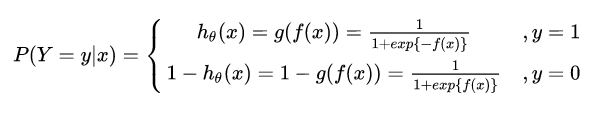将它带入到上式，通过推导可以得到logistic的损失函数表达式，如下：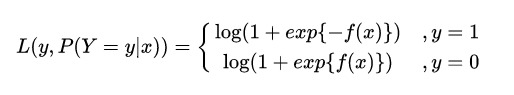逻辑回归最后得到的目标式子如下：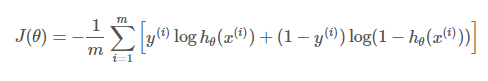上面是针对二分类而言的。这里需要解释一下：之所以有人认为逻辑回归是平方损失，是因为在使用梯度下降来求最优解的时候，它的迭代式子与平方损失求导后的式子非常相似，从而给人一种直观上的错觉。
这里有个PDF可以参考一下：Lecture 6: logistic regression.pdf.
注意：softmax使用的即为交叉熵损失函数，binary_cossentropy为二分类交叉熵损失，categorical_crossentropy为多分类交叉熵损失，当使用多分类交叉熵损失函数时，标签应该为多分类模式，即使用one-hot编码的向量。
二、平方损失函数（最小二乘法, Ordinary Least Squares ）
最小二乘法是线性回归的一种，最小二乘法（OLS）将问题转化成了一个凸优化问题。在线性回归中，它假设样本和噪声都服从高斯分布（为什么假设成高斯分布呢？其实这里隐藏了一个小知识点，就是中心极限定理，可以参考【central limit theorem】），最后通过极大似然估计（MLE）可以推导出最小二乘式子。最小二乘的基本原则是：最优拟合直线应该是使各点到回归直线的距离和最小的直线，即平方和最小。换言之，OLS是基于距离的，而这个距离就是我们用的最多的欧几里得距离。为什么它会选择使用欧式距离作为误差度量呢（即Mean squared error， MSE），主要有以下几个原因：

简单，计算方便；
欧氏距离是一种很好的相似性度量标准；
在不同的表示域变换后特征性质不变。

平方损失（Square loss）的标准形式如下：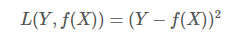当样本个数为n时，此时的损失函数变为：Y-f(X)表示的是残差，整个式子表示的是残差的平方和，而我们的目的就是最小化这个目标函数值（注：该式子未加入正则项），也就是最小化残差的平方和（residual sum of squares，RSS）。
而在实际应用中，通常会使用均方差（MSE）作为一项衡量指标，公式如下：上面提到了线性回归，这里额外补充一句，我们通常说的线性有两种情况，一种是因变量y是自变量x的线性函数，一种是因变量y是参数α的线性函数。在机器学习中，通常指的都是后一种情况。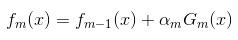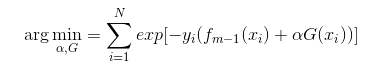而指数损失函数（exp-loss）的标准形式如下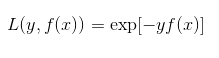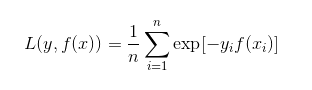四、Hinge损失函数（SVM）
在机器学习算法中，hinge损失函数和SVM是息息相关的。在线性支持向量机中，最优化问题可以等价于下列式子：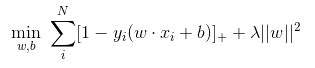下面来对式子做个变形，令：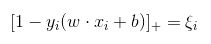于是，原式就变成了：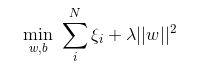如若取λ=1/(2C)，式子就可以表示成：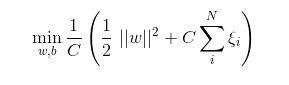可以看出，该式子与下式非常相似：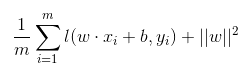前半部分中的 l 就是hinge损失函数，而后面相当于L2正则项。
Hinge 损失函数的标准形式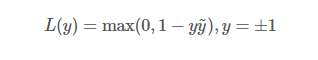可以看出，当|y|>=1时，L(y)=0。
更多内容，参考Hinge-loss。
补充一下：在libsvm中一共有4中核函数可以选择，对应的是-t参数分别是：

0-线性核；
1-多项式核；
2-RBF核；
3-sigmoid核。

五、其它损失函数
除了以上这几种损失函数，常用的还有：
0-1损失函数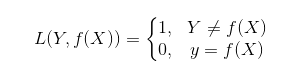绝对值损失函数下面来看看几种损失函数的可视化图像，对着图看看横坐标，看看纵坐标，再看看每条线都表示什么损失函数，多看几次好好消化消化。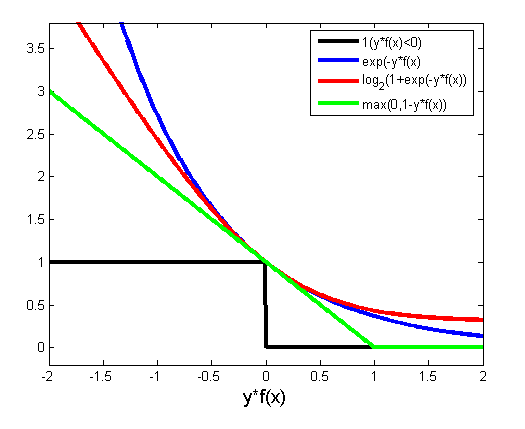六、Keras / TensorFlow 中常用 Cost Function 总结

mean_squared_error或mse

mean_absolute_error或mae

mean_absolute_percentage_error或mape

mean_squared_logarithmic_error或msle

squared_hinge

hinge

categorical_hinge

binary_crossentropy（亦称作对数损失，logloss）

logcosh

categorical_crossentropy：亦称作多类的对数损失，注意使用该目标函数时，需要将标签转化为形如(nb_samples, nb_classes)的二值序列

sparse_categorical_crossentrop：如上，但接受稀疏标签。注意，使用该函数时仍然需要你的标签与输出值的维度相同，你可能需要在标签数据上增加一个维度：np.expand_dims(y,-1)

kullback_leibler_divergence:从预测值概率分布Q到真值概率分布P的信息增益,用以度量两个分布的差异.

poisson：即(predictions - targets * log(predictions))的均值

cosine_proximity：即预测值与真实标签的余弦距离平均值的相反数

需要记住的是：参数越多，模型越复杂，而越复杂的模型越容易过拟合。过拟合就是说模型在训练数据上的效果远远好于在测试集上的性能。此时可以考虑正则化，通过设置正则项前面的hyper parameter，来权衡损失函数和正则项，减小参数规模，达到模型简化的目的，从而使模型具有更好的泛化能力。
原文链接


展开全文• 没有一个适合所有机器学习算法的损失函数。针对特定问题选择损失函数涉及到许多因素，比如所选机器学习算法的类型、是否易于计算导数以及数据集中异常值所占比例。 文章目录均方误差/平方损失/L2 损失(MSE)平均绝对...深度学习
• 写在前面：一定要分清楚损失函数与误差函数！ 损失函数：是指训练时候预测值与真实值的误差函数，我们应用凸优化,SGD等来使它减小，得到一些模型参数！ 误差函数：是指模型训练完后，用测试集数据喂进去模型得到的...
• 看到一篇关于损失函数简单且逻辑清晰的文章，转载一下，赶紧博主总结的很简单易懂，很棒。 基本概念： 损失函数：计算的是一个样本的误差 代价函数：是整个训练集上所有样本误差的平均 目标函数：代价函数 + ...
• 今天这篇文章和大家聊聊机器学习领域的熵。我在看paper的时候发现对于交叉熵的理解又有些遗忘，复习了一下之后，又有了一些新的认识。故写下本文和大家分享。熵这个概念应用非常广泛，我个人认为比较经典的一个应用...
• 1）均方误差（mean squared error） 程序实现 def mean_squared_error(y, t): return 0.5 * np.sum((y-t)**2) 举例： t = [0, 0, 1, 0, 0, 0, 0, 0, 0, 0]#假设第二个位置为正确值，ont-hot显示 ...
• 损失函数（loss function）是用来估量模型的预测值$$f(x)$$与真实值$$Y$$的不一致程度，从而衡量模型预测的好坏。它是一个非负实值函数,通常使用$$L(Y, f(x))$$来表示，损失函数越小，模型的鲁棒性就越好。 常用损失...
• 我们学习一个算法总是要有个指标或者多个指标来衡量一下算的好不好，不同的机器学习问题就有了不同的努力目标，今天我们就来聊一聊回归意义下的损失函数、正则化的前世今生，从哪里来，到哪里去。 一．L1、L2下的...正则化 Lasso
• 损失函数总结(适用算法) 1. 对数损失函数 （朴素贝叶斯，EM，HMM） L(Y , P(Y | X)) = -log P(Y | X) 2. 平方损失函数（最小二乘法） L(y , f(x)) = 〖(y – f(x))〗^2 3. 指数损失函数（AdaBoost） L(y , f(x)) = ...
• 交叉熵可在神经网络(机器学习)中作为损失函数，p表示真实标记的分布，q则为训练后的模型的预测标记分布，交叉熵损失函数可以衡量p与q的相似性。    交叉熵作为损失函数还有一个好处是使用sigmoid函数在梯度...
• 损失函数(Loss/Error Function): 计算单个训练集的误差，例如：欧氏距离，交叉熵，对比损失，合页损失 代价函数(Cost Function): 计算整个训练集所有损失之和的平均值 至于目标函数(Objective function)，字面一些...
• 详解机器学习中的损失函数0.6872018.08.13 16:39:22字数 2217阅读 757 Class Content layout Blog title 详解机器学习中的损失函数 categories Blog description 机器学习中常见的损失函数以及它们的...
• 机器学习常见损失函数 损失函数作用：损失函数是用于衡量模型预测值与真实值之间差距的函数，损失函数的值越小越好。 常见的损失函数有如下几种： 分类问题 0-1损失函数（Zero-one Loss）： 当且仅当预测为真的时候...深度学习 人工智能
• 目标函数是最小化损失函数损失函数：，损失函数越小，拟合的越好。 风险函数：是损失函数的期望，输入与输出遵循联合分布，但是分布未知。可以用训练集来参考，训练集的平均损失成为经验风险，即,最小化经验...
• 有这样一个问题，损失函数和评价指标都...损失函数各种各样，没有哪一个说适合所有的网络模型，损失函数的选取依赖于参数的数量、异常值、机器学习算法、梯度下降的效率、导数求取的难易和预测的置信度等若干方面。 损
• 机器学习——损失函数前言一、分类问题的损失函数1、0-1损失（one-zero loss）2、Log Loss3、Focal Loss4、相对熵、KL散度（Relative Entropy/Kullback-Leibler Divergence）5、指数损失（Exponential Loss）6、......